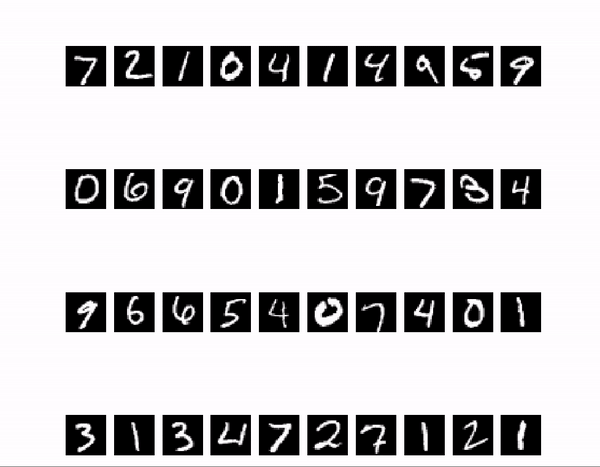# 1.First of all

I was able to evaluate the model with Tensorflow, PyTorch, and Chainer, but isn't it possible to show only the Accuracy and Loss graphs to people who are not familiar with Deep Learning? Also, this image is the correct answer quickly! This is incorrect! There will be some scenes where you will be happy to see that. In order to fulfill such a wish, I would like to realize with Matplotlib that the judgment result will be posted on multiple images arranged side by side.

"--- A happy boy. Your wish will finally come true."Also, this time we will use Tensorflow as an example, but the display part of the image can be any framework.

# 2. Implementation & Description

As an example, I prepared a model that trained MNIST using Tensorflow. This time, 40 images will be used.

#### `validation.py`

``````
from matplotlib import pyplot as plt
import numpy as np
import tensorflow as tf

#Setting the number of display images
row = 4
col = 10

mnist = tf.keras.datasets.mnist
(_, _), (x_test, y_test) = mnist.load_data()
x_test = np.asarray(x_test[0:row*col])
y_test = np.asarray(y_test[0:row*col])

path = 'mnist.h5' #Trained model path

#inference
x_test_flat = x_test.reshape(-1, 784) / 255.0
result = model.predict(x_test_flat)

#Image alignment
plt.figure(figsize=(5, 5))
image_array = []
for i, image in enumerate(x_test):
image_array.append(plt.subplot(row, col, i + 1))
plt.axis('off')
plt.imshow(image, cmap='gray')
plt.pause(0.1)

#Label placement
for j, image in enumerate(x_test):
bg_color = 'skyblue' if y_test[j] == np.argmax(result[j]) else 'red'
image_array[j].text(0, 0, np.argmax(result[j]), color='black', backgroundcolor=bg_color)
plt.pause(0.1)

#Save the entire image
plt.savefig('judge_result.png')
``````

It is written on various sites up to the point of learning and evaluating and outputting a graph of recognition rate. However, it is surprisingly few that it is written that inference is done with the learned model. (Although it is my own experience ...)

``````x_test_flat = x_test.reshape(-1, 784) / 255.0
result = model.predict(x_test_flat)
``````

`tensorflow.keras.models` has a method called`predict ()`, which is used for inference. Pass this method an array of images you want to infer. Since the input of the model is one-dimensional, I converted it to a one-dimensional array with `reshape (-1, 784)`. Since we are processing 40 sheets at a time this time, we will pass an array of `(40, 784)`, but when processing only one sheet, we need to pass it as `(1, 784)`.

In Chainer, inference is possible (should be) by writing `result = model.predictor (test_x) .data `.

With matplotlib, you can write in an object-oriented manner.

``````plt.figure(figsize=(5, 5))
image_array = []
for i, image in enumerate(x_test):
image_array.append(plt.subplot(row, col, i + 1))
plt.axis('off')
plt.imshow(image, cmap='gray')
plt.pause(0.05)
``````

In the first for statement, use `plt.subplot` to arrange the images. `plt.subplot` is a child element of` figure`. Pass (vertical, horizontal, number) as an argument. The number that represents the number is counted from 1. (Note that it is not from 0) First, display all the images, and then add labels to the child elements, so let's append () `so that you can operate them. (If you just want to display it, you don't need to put it in the array, but I want to add a label later, so I will do it this time.) Also, since this time it is an image, not a graph, the display of coordinates is turned off with `plt.axis ('off')`. When you have finished preparing the images to be arranged at once, display the images with `plt.pause ()`. If you set it to `plt.show ()`, the process will stop there, so I use `plt.pause ()`.

``````for j, image in enumerate(x_test):
bg_color = 'skyblue' if y_test[j] == np.argmax(result[j]) else 'red'
image_array[j].text(0, 0, np.argmax(result[j]), color='black', backgroundcolor=bg_color)
plt.pause(0.05)
``````

The second for statement adds labels to the elements of ʻimage_array` one by one. Add the inference result to the image with ʻimage_array [j] .text (0, 0, np.argmax (result [j])`. If the inference result `np.argmax (result [j])` and the correct label `y_test [j]` match, the background is blue, and if they do not match, the background is red. Display the label on the screen with `plt.pause ()`. The argument is the time to display the image, and changing this value will change the display update speed. Please note that it is the update speed of "display", not the processing speed of the model.

# 3. Summary

I made this one, but I wanted to use it in the presentation of the student experiment, but I couldn't implement it at that time ... It's easy to think about now, but it can be difficult depending on the learning stage. I hope it reaches people who have just started deep learning or who have taken programming lectures but dislike it and do not understand it very well.